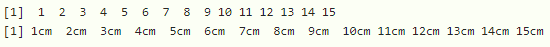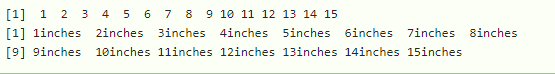How to Create a Unit Object with the grid Package in R

• Last Updated : 31 Aug, 2021

In this article, we are going to discuss how to create a unit object with a grid package in R programming language.

The unit describes the quantity of particular data present in a vector/dataframe/list. Here we will get data units in required formats using the unit() function. It is available in the grid package, thus it has to be imported to the workspace.

Syntax:

library(“grid”)

we can get the units by using unit() function

Syntax:

unit(data,”unit_name”)

where

• data is the input data
• unit_name is the type of unit

Given below are implementations for the same.

Example:  R program to create a vector with 15 values and display units in centimeter

R

 # import grid package library("grid")    # vector with 15 values vec=c(1:15)    # display vector print(vec)    # get the units in cm print(unit(vec,"cm"))

Output:Example: R program to get the units in inch

R

 # import grid package library("grid")    # vector with 15 values vec=c(1:15)    # display vector print(vec)    # get the units in inches print(unit(vec,"inch"))

Output:My Personal Notes arrow_drop_up
Recommended Articles
Page :